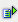The function left (string, num) returns the first (num) characters of a text string.

Syntax: =left(string,num)Example Cell A1 = abcdefgh =left(A1,3) is abc =left(A1,5) is abcde